Properties of Triangles
edHelper subscribers - Create a new printable

Number of Keys
 Select the number of different printables: 1 key 2 keys 3 keys 4 keys 5 keys

Number of Pages (for each key)
 Select the number of pages: 1 page 2 pages 3 pages 4 pages

Include an answer key (answer keys will be at the end of the printable)
High School Geometry
High School Geometry
Math

 Name _____________________________Date ___________________ (Key 1 - Answer ID # 0325036)
1.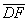and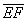bisect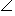ADE andCED respectively. If mCED = 113and mDFE = 69, find mABF.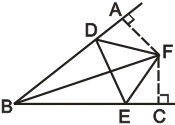2.

The perpendicular bisectors of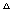ABC meet at point G. If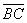= 16,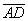= 5, and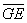= 3, find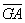.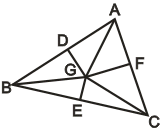3.

InABC,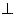and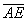bisectsBAC, mB = 74, and mC = 32. Find mDAE.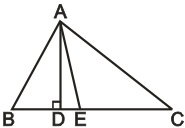4.

Which of the following statements is NOT correct?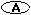In an isosceles triangle, the altitude to the base bisects the base and the vertex angle.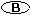An equilateral triangle is also an equiangular triangle.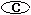The shortest distance from a vertex to the opposite side is the altitude to that side.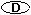The circumcenter of a triangle is always inside the triangle.

5.

The governor wants to build a new library for three cities X, Y, and Z. If the distance between each two cities is 20 kilometers, and the location of the new library will be in equidistance to all three cities, what is the distance between the new library and city X?

6.

You want to prove that the perpendicular bisector of the base of an isosceles triangle is also the angle bisector of the vertex. Which postulate can NOT be used in your proof?SSAHLSASSSS

Properties of Triangles
edHelper subscribers - Create a new printable

Number of Keys
 Select the number of different printables: 1 key 2 keys 3 keys 4 keys 5 keys

Number of Pages (for each key)
 Select the number of pages: 1 page 2 pages 3 pages 4 pages